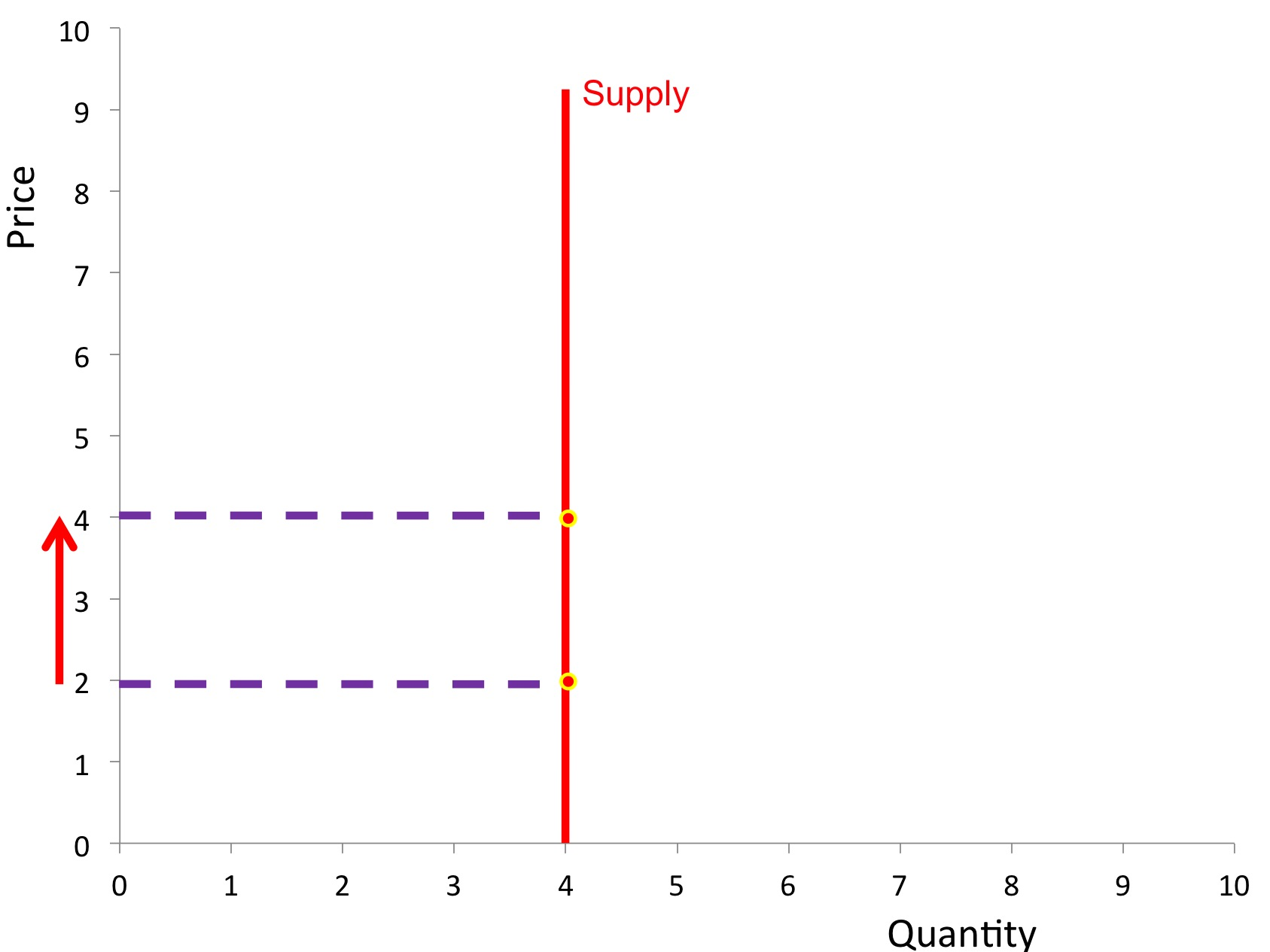#cfa #economics #has-images

A perfectly inelastic supply curve, with elasticity value of zero, means that the quantity supplied doesn’t change irrespective of the level of demand.If you want to change selection, open document below and click on "Move attachment"

Elasticity of Supply - Revisionguru
and, there are special cases of elasticity of supply. If elasticity is equal to one then the good will have unitary elasticity; meaning a percentage change in price will lead to an equal percentage change in the quantity supplied. <span>A perfectly inelastic supply curve, with a value of zero, means that the quantity supplied doesn’t change irrespective of the level of demand. Elasticity of supply is equal to infinity when it is perfectly elastic; any decrease in price will lead to the quantity supplied falling to zero.